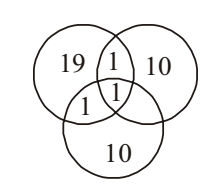Home » Verbal Reasoning » Logical Venn Diagram » Question

#### Logical Venn Diagram

1. In a group of persons, 11 persons speak Kannada, 20 persons speak Tamil and 11 persons speak Telugu. In that group, if two persons speak 2 languages and 1 person speak all the languages, then how many persons are there in the group?1. 40
2. 41
3. 42
4. 43
##### Correct Option: C

According to question ,
Let us assume Number of Kannada = K , Number of Tamil = T and Number of Telugu = Te
Here , n(K) = 11; n(T) = 20; n(Te) = 11
n(K ∩ T ∩ Te) = 1
n(K ∩ T) = n(T ∩ Te) = n(Te ∩ K) = 2
n(K U T U Te) = n(T) + n(K) + n(Te) - n(K ∩ T) - n(T ∩ Te) - n(Te ∩ K) + n(K ∩ T ∩ Te)
n(K U T U Te) = 20 + 11 + 11 - 2 - 2 - 2 + 1 = 37